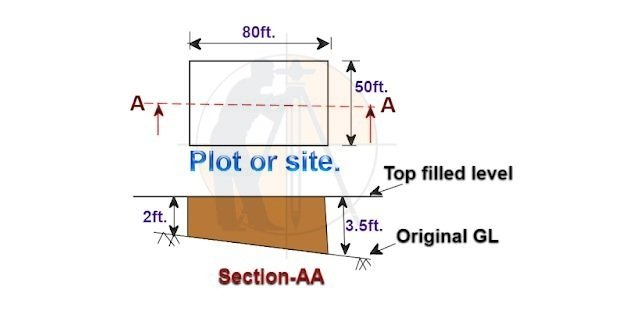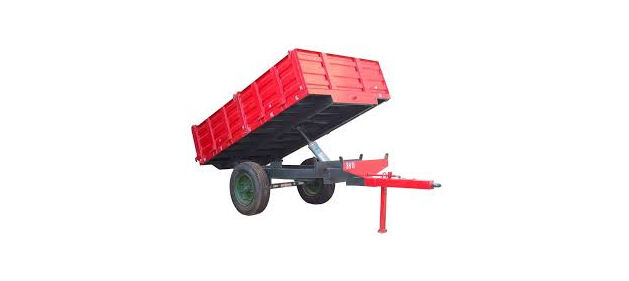Breaking News
Home / Civil Engineering / Calculate The Cost Of Filling A Plot With Construction Soil

# Calculate The Cost Of Filling A Plot With Construction Soil

### Calculate The Cost Of Filling A Plot With Construction Soil (moo-rum)?

Let us consider a Plot or Site  as shown in the below drawing for the calculation purpose…Given data:

Length of the plot = 80 ft.

The breadth of the plot = 50 ft.

Depth of the plot to be filled at front end = 2 ft.

Depth of the plot to be filled at back end = 3.5 ft.

The volume of the plot to be filled with construction soil.

= [length × breadth × (average depth of the plot to be filled)]

= [ 80 ft. × 50 ft. × {( 2ft. + 3.5 ft.) ÷ 2}]

=  [ 80 ft. × 50 ft. × 2.75 ft.]

= 11,000 cu ft.

### What Is Difference Between P.P.C And O.P.C Cement

When we fill the plot with the loose soil, we need an extra volume of soil for settlement & compaction.

As you know,

Loose soil = 1.25 × compacted soil.

So, the volume of soil required to fill the plot

= 1.25 × 11,000 cu ft.

13, 750 cu ft.

389.36 cum.

( As 1 cum. = 35.3147 cu ft.)

The cost of filling:

The cost of construction soil falls in a range of INR 300/- to 500/– per cum.

Let us consider an average of INR 400/– for the calculation purpose.

The cost of filling the plot with soil

= [vol. of soil required in cum. × cost / cum.]

= [ 389.36 cum. × 400/- per cum.]

INR 1,55,744/-

The soil required in no. of trucks:

The vol. of a truck container = 500 cu ft.

The no. of truck required

= [ the vol. of soil required in cu ft. ÷ the vol. of a truck]

= [ 13,750 cu ft. ÷ 500 cu ft.]

27.5 nos.

The soil required in no. of tractor-trolley:The vol. of a trolley = 70 cu ft.

The no. of trolley required

= [ the vol. of soil required in cu ft. ÷ the vol. of a trolley]

= [ 13,750 cu ft. ÷ 70 cu ft.]

= 196.43 nos. say 197 nos.

Note:

1. The cost of construction soil varies according to the regional market rate. Replace the soil cost to get the correct results.

2. Here, the compaction factor of 1.25 is taken, by considering the natural compaction by rainwater & vehicle movements. If the roller or mechanical compactor is used, the multiplication factor will be 1.35.

## . Land Surveying & Architects

### THANKS.